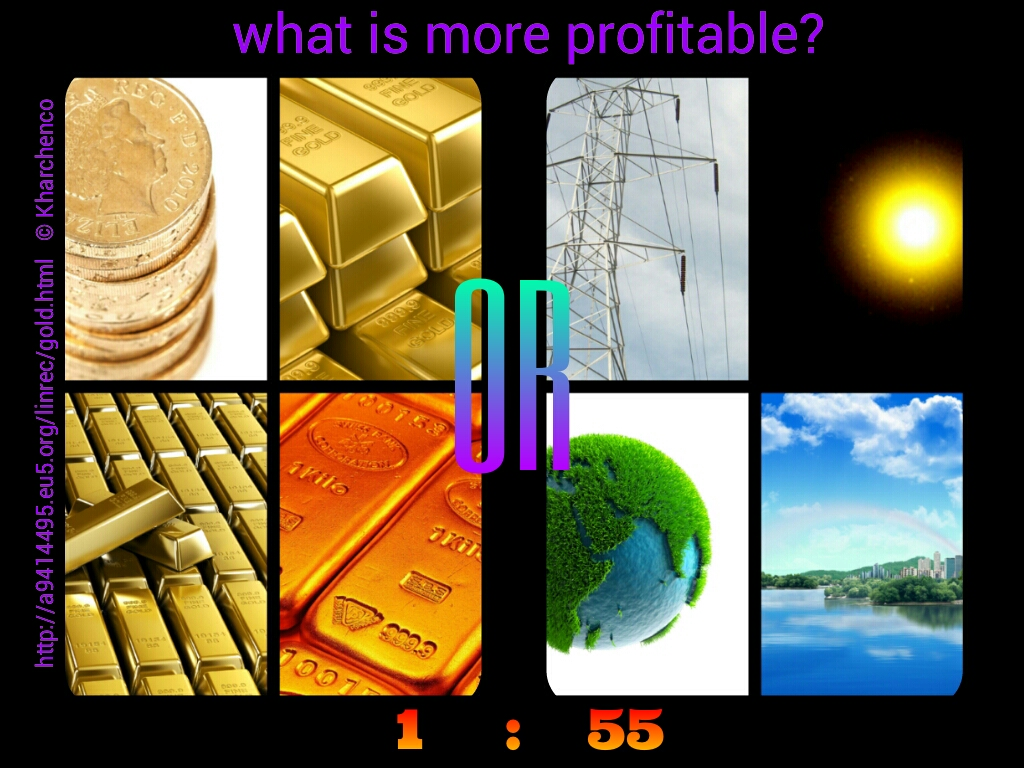## LINEAR FUSION REACTOR LINRECSince ancient times alchemists looked for the "philosopher's stone", capable to turn simple metal into gold. Now nuclear reactions in accelerators allow to receive gold from mercury or lead. However, expenses on receiving such artificial gold are much higher than its market cost.

But even if it would be succeeded to receive gold from lead by means of philosopher's stone without expenses, it would be not the most profitable business and not the best investment. It appears that known fusion reaction of a deuterium with tritium (D+T—>He+n+17.6MeV) yields revenue many times more, than receiving gold from lead by means of alchemy.

Let's compare the income from receiving gram of gold and from obtaining energy as a result of reaction of one gram of a deuterium with tritium.

Let's count, what income will give receiving one gram of gold from lead (without any expenses). The cost of gram of gold from http://goldpricenetwork.com/gram/ makes \$50.75 (9.03.2013).

Now we will calculate the income from receiving the electric power at reaction of one gram of a deuterium with tritium. The average cost of one kilowatt-hour of the electric power for the consumer makes about \$0.12 ( http://epp.eurostat.ec.europa.eu/statistics_explained/index.php/Energy_price_statistics , http://michaelbluejay.com/electricity/cost.html ). Let's accept that wholesale price of sale by the producer of 1 kilowatt-hour of the electric power makes about 33% from the price for the consumer, then the income of the producer from sale of 1 kilowatt-hour makes p=\$0.04. At reaction of 1 kernel of a deuterium with tritium energy of E=17.6MeV is emitted. Then, E=17.6*10^6*1.60217646*10^(-19)[it is an electron charge]/(3,6*10^6) [number of joules in kilowatt-hour]=7.83286269*10^(-19) kilowatt-hours.

Now we will count, how many kernels are there in 1 gram of a deuterium. The mass of one atom of a deuterium 2.0141017778[atomic weight in atomic mass unit]*1.66053886*10^ (-27)kg=3.34449427*10^(-27)kg. Number of kernels of a deuterium in one gram is N=10^(-3)/(3.34449427*10^(-27))=2.9899887*10^23. At reaction of one gram of a deuterium with tritium energy of Q=E*N=7.83286269*10^(-19)kWh*2.9899887*10^23=2.34201709*10^5kWh is emitted. Now this heat should be turned into the electric power. Average efficiency of this process is 30% ( http://www.mpoweruk.com/energy_efficiency.htm). Then at reaction of 1 gram of a deuterium we receive 2.34201709*10^5kWh*0.3 = 0.70260513*10^5kWh of electric energy, that will bring in the income of \$0.04*0.70260513*10^5=\$2810.421. This income costs 2810.421/50.75=55.3777537 (approximately 55) TIMES MORE than PURE GOLD.

So, the income from reaction of one gram of a deuterium with tritium costs 55 times more than income from receiving gold from lead by means of alchemy. Even if to accept that all expenses in receiving reaction of a deuterium with tritium in the real reactor make 95%, and the net profit makes 5%, even in this case the net profit will be in 55/20=2.75 times more than profits on receiving pure gold without expenses. Is it "philosopher's stone", isn't it? :-) :-) :-)

So milliard expenses on ITER, investments of the eminent companies in researches on thermonuclear fusion and all subsequent expenses on construction of experimental reactors will pay off a hundredfold. Not to mention that thermonuclear fusion is the most real rescue from an ecological disaster.

## Linear Fusion Reactor Linrec